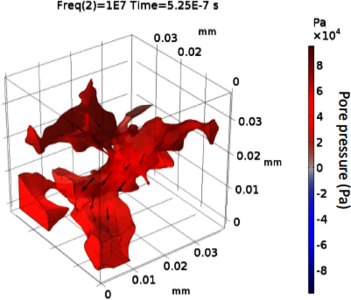# 科技论文和技术资料

#### Numerical Simulation of Coupled Fluid-Solid Interaction in Digital Rock Samples

V. Das , T. Mukerji , G. Mavko ,
 Stanford University, Stanford, CA, USA

Digital rock physics (DRP) is an emerging field where a rock sample is imaged, relevant physical processes are simulated numerically on the digital rock sample, and the numerical solutions are used for understanding and interpreting the rock in different in-situ conditions. The use of DRP in obtaining realistic measurements comparable to conventional laboratory measurements hence depends on many factors. One key factor is our ability to simulate rigorous physical processes at the pore scale. In this work, we use the COMSOL Multiphysics® software to perform numerical modeling to capture the physics related to coupled fluid-solid interaction (FSI) and the frequency-dependence of pore-scale fluid flow in response to pore pressure heterogeneities. Previously, FSI in the digital rocks are modeled using approximations that either consider the fluid as an elastic/ viscoelastic material or use simplified 2D geometries. In this work, we consider the solid grain as linear isotropic elastic material with elastic moduli of quartz and the pore fluid as compressible Newtonian fluid with material properties of heavy oil. We solve the equation of motion along with the constitutive law given by Hooke’s law for the stresses and strains in the grain using the Solid Mechanics interface in COMSOL®. For the pore fluid, we compute the pressure and velocity fields by solving the compressible form of the Navier-Stokes’ equation using the Laminar Flow interface in COMSOL®. We set displacement boundary conditions like a P-wave modulus experimental setup in the lab. We apply a monochromatic time-dependent sinusoidal displacement at the top boundary. The bottom surface is fixed, and the side surfaces have roller boundary conditions. The FSI coupling at the interface between the grain and the pore is done using the Fluid-Structure Interaction Multiphysics interface in COMSOL®. The coupling is done by setting the fluid velocity at the interface equal to the solid velocity. We simulated the physics on a micro-CT scan of Berea sandstone, that was imported into COMSOL® and meshed with tetrahedral elements, with finer elements in the pore space, to capture the pore scale physics more accurately. The total number of mesh elements is ~327000, and the total degrees of freedom solved is ~1245000. We used the time-dependent study with fully-coupled iterative geometric multigrid solver in COMSOL® to solve the time-dependent initial boundary value problem. Parametric sweep in COMSOL® was used to perform the frequency dependent simulations at various frequencies of the applied displacement at the top boundary.
The numerical results show that at low frequencies (10 KHz), the pore pressure is in equilibrium. However, at high frequencies (10 MHz), the pore pressure is heterogeneous. At even higher frequencies (100 MHz), we observe scattering and resonance effects that lead to high pore pressure heterogeneities. Numerical simulations of the dynamic fluid effects in the digital rock samples will help in bridging the gap between seismic measurements (surface seismic, sonic logs and ultrasonic lab measurements) taken at different frequencies. The stress and pore pressure distribution that can only be obtained from numerical simulations can also be useful for engineering applications like enhanced oil recovery.